GATE  >  Test: Engineering Mathematics & General Aptitude- 2

# Test: Engineering Mathematics & General Aptitude- 2

Test Description

## 25 Questions MCQ Test GATE Civil Engineering (CE) 2023 Mock Test Series | Test: Engineering Mathematics & General Aptitude- 2

Test: Engineering Mathematics & General Aptitude- 2 for GATE 2023 is part of GATE Civil Engineering (CE) 2023 Mock Test Series preparation. The Test: Engineering Mathematics & General Aptitude- 2 questions and answers have been prepared according to the GATE exam syllabus.The Test: Engineering Mathematics & General Aptitude- 2 MCQs are made for GATE 2023 Exam. Find important definitions, questions, notes, meanings, examples, exercises, MCQs and online tests for Test: Engineering Mathematics & General Aptitude- 2 below.
Solutions of Test: Engineering Mathematics & General Aptitude- 2 questions in English are available as part of our GATE Civil Engineering (CE) 2023 Mock Test Series for GATE & Test: Engineering Mathematics & General Aptitude- 2 solutions in Hindi for GATE Civil Engineering (CE) 2023 Mock Test Series course. Download more important topics, notes, lectures and mock test series for GATE Exam by signing up for free. Attempt Test: Engineering Mathematics & General Aptitude- 2 | 25 questions in 75 minutes | Mock test for GATE preparation | Free important questions MCQ to study GATE Civil Engineering (CE) 2023 Mock Test Series for GATE Exam | Download free PDF with solutions
 1 Crore+ students have signed up on EduRev. Have you?
*Answer can only contain numeric values
Test: Engineering Mathematics & General Aptitude- 2 - Question 1

### Determine the minimum number of vertices to be removed from a complete graph K10 to make the graph disconnected.

Test: Engineering Mathematics & General Aptitude- 2 - Question 2

### Which of the following propositions is/are a contingency?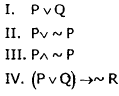Detailed Solution for Test: Engineering Mathematics & General Aptitude- 2 - Question 2

A contingency is a proposition that is neither a tautology nor a contradiction.

Test: Engineering Mathematics & General Aptitude- 2 - Question 3

### The number of one-one (injective) functions from a set of 7 elements to a set of 5 elements is

Detailed Solution for Test: Engineering Mathematics & General Aptitude- 2 - Question 3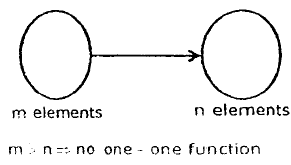Test: Engineering Mathematics & General Aptitude- 2 - Question 4

Direction : This question consists of a word or a phrase that is underline. It is followed by four words or phrases. Select the word or phrase that is most closely opposite in meaning to the underline word or phrases

Q.

The teachers have been.observing his impertinent behaviour.

Test: Engineering Mathematics & General Aptitude- 2 - Question 5

Direction : In the given below sentence, each sentence has three parts, indicated by (a), (b), (c). Read sentence to find out whether there is any error. If you find any error in any one part (a, b, c) indicate your response by blackening the letter related to the part in the answer sheet provided. If a sentence has no error, indicate this by blackening (d) which stands for ‘no error'.

Q.

He goes / (a)  to college / (b) by foot everyday / (c) No error (d)

Detailed Solution for Test: Engineering Mathematics & General Aptitude- 2 - Question 5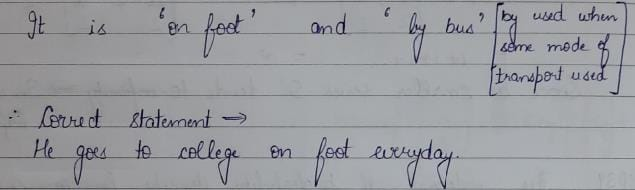Test: Engineering Mathematics & General Aptitude- 2 - Question 6

Direction : In the following question, sentence is given with blank to be filled with an appropriate word(s). Four alternatives are suggested, choose the correct alternative

Q.

However honest he____________________ I do nottrust him.

Test: Engineering Mathematics & General Aptitude- 2 - Question 7

In this question a related pair of word of followed by four pair of words. Select the pair that best expresses a relationship similar to the expressed in original pair.

MARATHON : STAMINA

Test: Engineering Mathematics & General Aptitude- 2 - Question 8

A shopkeeper gives 12% additional discount on the discounted price, after giving an initial discount of 20% on the labelled price of a radio. If the final sale price of the radio is Rs. 704, then what is its labelled price?

Detailed Solution for Test: Engineering Mathematics & General Aptitude- 2 - Question 8

Let the marked price be Rs. 100.

Successive discounts of 20% and 12% were given.

Sale price = 100*80100*88100=Rs.704/10

If the sale price is Rs.704/10, the marked price is Rs.100

If the sale price is Rs.704, the marked price is

= 100*10*704/704= Rs.1000

Test: Engineering Mathematics & General Aptitude- 2 - Question 9

Find the odd man out

385, 462, 572, 396. 427, 671,264

*Answer can only contain numeric values
Test: Engineering Mathematics & General Aptitude- 2 - Question 10

25 persons are in a room. 15 of them play hockey, 17 of them play football and 10 of them play both hockey and football. Then the number of persons playing neither hockey nor football is

Detailed Solution for Test: Engineering Mathematics & General Aptitude- 2 - Question 10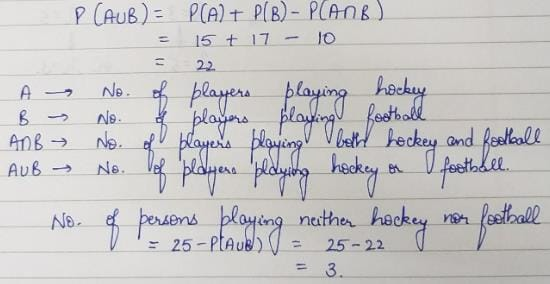Test: Engineering Mathematics & General Aptitude- 2 - Question 11

In a factory, a radioactive source emits 2 particles (on an average) per second. The probability that 2 or more particles will be emitted in an interval of 5 seconds is

Detailed Solution for Test: Engineering Mathematics & General Aptitude- 2 - Question 11

X = 2.0
The number of particles emitted in the interval of 5 seconds is Poisson distributed with parameter = 5λ = 10
The required probability is, P(x > 2) = 1-P(x < 2) = 1 - P(x = 0) -P (x = 1) = 1 - e-10 -10e-10 = 1-11e-10

Test: Engineering Mathematics & General Aptitude- 2 - Question 12

Consider the set of all integers (Z) with the operation defined as m*n = m + n + 2, m,ne Z

Q.

If (z, *) forms a group, then determine the left identity element.

Detailed Solution for Test: Engineering Mathematics & General Aptitude- 2 - Question 12

(Z, *) forms a group. Let e ∈ z be the left identity element.
Then e * m = m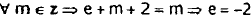Test: Engineering Mathematics & General Aptitude- 2 - Question 13

Consider the set of all integers (Z) with the operation defined as m*n = m + n + 2, m,ne Z

Q.

Determine the left inverse of element (-4)

Detailed Solution for Test: Engineering Mathematics & General Aptitude- 2 - Question 13

Let m ∈ z and m' ∈ zbe the left inverse of element m
Then m’* m = e m’+ m + 2 = -2 ⇒ m` = -m- 4
Here m = (-4) ∴ m` = 0
and m'* m = 0*(-4) = 0-4 +2 =-2 = e

Test: Engineering Mathematics & General Aptitude- 2 - Question 14

Consider the following probability distribution table of a discrete random variable X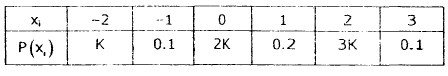Q.

Find the value of K

Detailed Solution for Test: Engineering Mathematics & General Aptitude- 2 - Question 14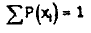⇒ K+0.1 +2K +0.2 +3K +0.1 = 1 ⇒ 6K + 0.4 = 1 ⇒ 6K = 0.6 => K = 0.1

Test: Engineering Mathematics & General Aptitude- 2 - Question 15

Consider the following probability distribution table of a discrete random variable XQ.

Calculate the Variance (V)

Detailed Solution for Test: Engineering Mathematics & General Aptitude- 2 - Question 15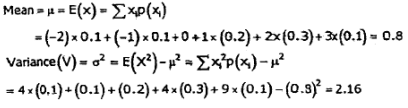Test: Engineering Mathematics & General Aptitude- 2 - Question 16

If DEER = 12215 and HIGH = 5645. how will you code HEEL?

Test: Engineering Mathematics & General Aptitude- 2 - Question 17

In a competitive examination in State A, 6% candidates got selected from the tota appeared candidates. State B had an equa number of candidates appeared and 7% candidates got selected with 80 mom candidates got selected than A. What was the number of candidates appeared from each State?

Test: Engineering Mathematics & General Aptitude- 2 - Question 18

A sum of money is borrowed and paid back in two annual instalments of Rs. 882 each allowing 5% compound interest. The sum borrowed was

Test: Engineering Mathematics & General Aptitude- 2 - Question 19

A walks around a circular field at the rate of one round per hour while B runs around it at the rate of six rounds per hour. They start in the same direction from the same point at 7.30 a.m. They shall first cross each other at:

Test: Engineering Mathematics & General Aptitude- 2 - Question 20

Five friends A, B, C, D and E are standing in a row facing South but not necessarily in the same order. Only B is between A and E, C is immediate right to E and D is immediate left to A. On the basis of above information, which of the following statements is definitely true?

Test: Engineering Mathematics & General Aptitude- 2 - Question 21

Five children were administered psychological tests to know their intellectual levels. In the report, psychologist pointed out that the child A is less intelligent than the child B. The child C is less intelligent than the child D. The child B is less intelligent than the child C and Child A is more intelligent than the child E. Which child is the most intelligent?

Test: Engineering Mathematics & General Aptitude- 2 - Question 22

Production of fertilizers by a Company (in 10000 tonnes) over the Years given in following bar graph: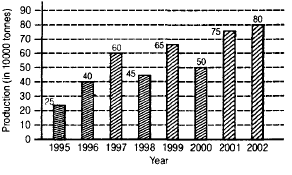In which year was the percentage increase in production as compared to the previous year the maximum?

*Answer can only contain numeric values
Test: Engineering Mathematics & General Aptitude- 2 - Question 23

These questions are to be answered on the basic ot the following pie chart which gives marks scored by a student in different subjects-English, Hindi, Mathematics, Science and Social Science in an examination. Assuming that the total marks obtained for the examination are 540 answer the following questions: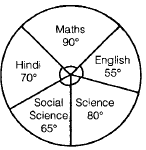The aggregate marks obtained by the students averaged over the 5 subjects is

Test: Engineering Mathematics & General Aptitude- 2 - Question 24

The following pie-chart shows the percentage of Literate and Illiterate-males and females in a city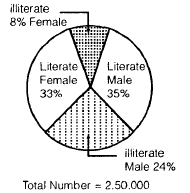What is the difference between the number of Literate Males and Literate Females?

Test: Engineering Mathematics & General Aptitude- 2 - Question 25

“P is son of Q, R, Q’s sister has a sons and daughter T. U is the maternal uncle of S. How is T related to U?

## GATE Civil Engineering (CE) 2023 Mock Test Series

26 docs|292 tests
 Use Code STAYHOME200 and get INR 200 additional OFF Use Coupon Code
Information about Test: Engineering Mathematics & General Aptitude- 2 Page
In this test you can find the Exam questions for Test: Engineering Mathematics & General Aptitude- 2 solved & explained in the simplest way possible. Besides giving Questions and answers for Test: Engineering Mathematics & General Aptitude- 2, EduRev gives you an ample number of Online tests for practice

## GATE Civil Engineering (CE) 2023 Mock Test Series

26 docs|292 tests